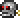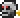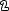•类型 \l!--\n --\g\{\{#vardefine:_typepage\!\{\{#or:\{\{l10n\!item_infobox\!typepage:@@@@\}\}\!\{\{tr\!\{\{ucfirst:@@@@\}\}\!link\ey\}\}\}\}\}\}\l!--\_\n --\g\{\{#vardefine:_typetext\!\{\{#or:\{\{l10n\!item_infobox\!type:@@@@\}\}\!\{\{tr\!\{\{ucfirst:@@@@\}\}\}\}\}\}\}\}\l!--\_\n --\g\{\{nowrap\!class\etag\!\{\{ifexists\!\{\{#var:_typepage\}\}\!\(\(\{\{#var:_typepage\}\}\!\{\{#var:_typetext\}\}\)\)\!\{\{#var:_typetext\}\}\}\}\}\}\l!--\n --\g\l!--\n --\g\{\{#vardefine:_typepage\!\{\{#or:\{\{l10n\!item_infobox\!typepage:@@@@\}\}\!\{\{tr\!\{\{ucfirst:@@@@\}\}\!link\ey\}\}\}\}\}\}\l!--\_\n --\g\{\{#vardefine:_typetext\!\{\{#or:\{\{l10n\!item_infobox\!type:@@@@\}\}\!\{\{tr\!\{\{ucfirst:@@@@\}\}\}\}\}\}\}\}\l!--\_\n --\g\{\{nowrap\!class\etag\!\{\{ifexists\!\{\{#var:_typepage\}\}\!\(\(\{\{#var:_typepage\}\}\!\{\{#var:_typetext\}\}\)\)\!\{\{#var:_typetext\}\}\}\}\}\}\l!--\n --\g ✔️ 45（很慢速度） 召唤机械骷髅王需要 3 份

使用 \{\{#lstmap:\$soundfiles\$\!,\!%%%%\!\l!--\_print\_sounds\n --\g\lspan\_style\e"margin-left:2px;"\g\{\{sound\!\!\{\{trim\!%%%%\}\}\}\}\l/span\g\l!--\n --\g\!\}\}

## 制作

total: 1 row(s)

### 用于

('"`UNIQ--esc-0000000D-QINU`"')

('"`UNIQ--esc-00000010-QINU`"')
total: 2 row(s)

## 花絮

• 骷髅头灯笼和机械骷髅头和骷髅最开始的外观十分相似：• 如同机械蠕虫一样，它看起来像是一个发条玩具。

## 历史

• 电脑版 1.2.1：掉率从0.1*1/1000 (0.1%)降低至0.04*1/2500 (0.04%)
• 电脑版 1.2
• 现在是一个困难模式掉落物。
• 制作不再需要铜锭。
• 制作所需的暗影之魂和光明之魂由 5 个降至 3 个。
• 主机版 1.02：现在是一个困难模式掉落物，并引入电脑版 1.2.1 的掉率。配方更改以符合电脑版 1.2。
• 移动版 1.2.6508：现在是一个困难模式掉落物，并引入电脑版 1.2.1 的掉率。配方更改以符合电脑版 1.2。